# Periodic Trends of Atomic Radii

## Introduction

Knowing the atomic radius helps us to predict the reactivity levels of atoms. The smaller the radii, they will be more reactive than the larger ones. This article covers all aspects of atomic radii of chemical elements. The periodic table patterns, different types of radii and so on, are discussed below.

## Define Atomic Radius and Atomic Size

The periodic table is an organized table for reference. It contains elements arranged with the smaller atomic number to the highest atomic number in different groups. The quantity of protons in the core of molecule is the atomic number. Atomic radius is defined as the measurement of the distance from the nucleus of an atom to the outermost electron available in the shell in isolated conditions. The atomic number from left to right of a periodic table increase progressively. But the atomic size in the periodic table decrease as you go from left to right. From top to down, both atomic number and size increase. Please refer to the figure below.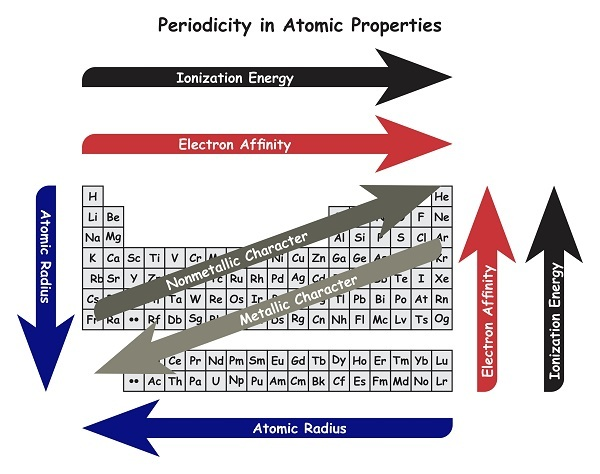Firstly, radius as a term is related to a geometrical figure circle. Secondly, atomic radius is characterized by approximating the appearance of an atom as a sphere. A sphere is a circle projected in the third dimension. How is the radius of a circle defined and how different is the atomic radius defined?

The diameter is a line between two points on the circle and passing through the center and radius it's half. An atom contains different shells in circle form, one over the other, and hence the radius of an atom is measured with the isolated electron in the outermost circle as portrayed below. Thus the separation from the center of the core to the exterior shell of an atom is called an atomic radius.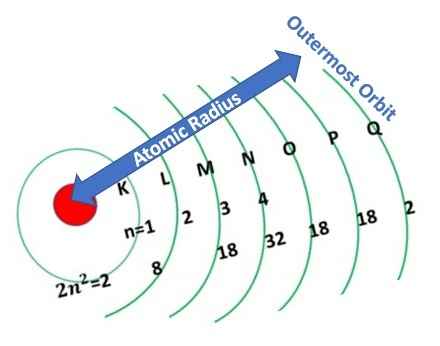There are many types of atomic radius concepts prevalent. As said earlier, it is difficult to exactly predict the shape and construct of an atom, due to limited facts available. The approximation of a circle or a sphere is one way. Let us see each of these concepts in detail below.

This concept is defined as the distance between the nucleus of two neighbouring atoms which are in non-bonded conditions. See how these two non-bonded atoms are themselves bonded into a compound molecule in the figure below.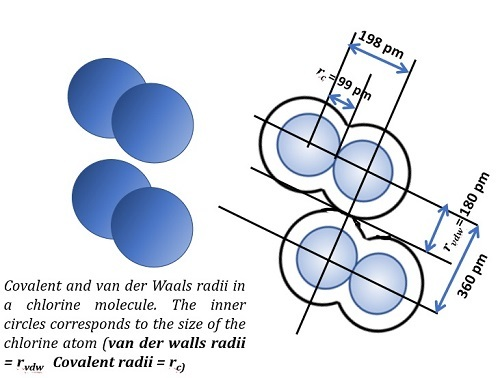The ionic bond is a type of electrovalent bond, where two oppositely charged ions attract each other to form a link. This bond exists not in an elemental state but in a chemical compound state. The distance between the nucleus of a positive ion and the nucleus of a negative ion is considered as twice the radius of an ion.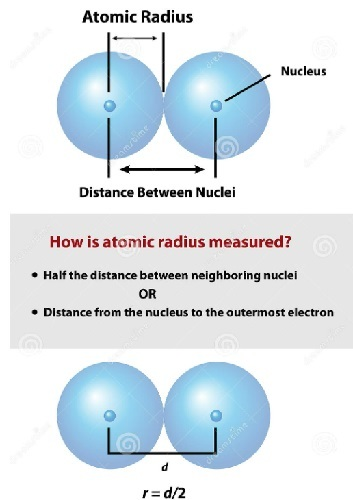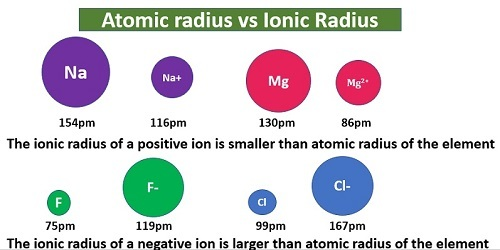In the scenario of covalent bonds also, the atomic radii concept remains the same, except that the chemical compound is formed by sharing electrons.

Here again, the metallic cluster is the differentiating element. Rest all remains the same. The binding of atoms in a metallic cluster is totally different from that of ionic binding formed by linking opposite polarity ions or covalent binding formed by sharing electrons. A concentrated structure of positive ions shares loosely spread electrons in metallic bonding. So metallic radius is half of the bond length between two metal ions.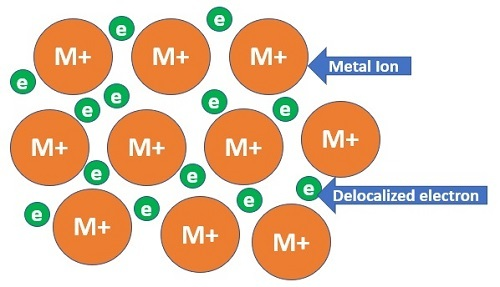## Difference Between Atomic Size and Atomic Radius

Atomic size is the size of an atom. Using the measurement of radius, we can conceive the atomic size. There are four methods available to compute the radius. So, in all fair sense, let us understand the differences between these four methods and relate them to atomic size.

1Measurement with bonded or non-bonded atoms?Non-bonded BondedBondedBonded positive ions
2Type of bondingWeak force between atomsAttractive force between positive and negative ionsBonding by sharing valence electronsBonding by metallic positive ions cluster and free electrons
3Bond lengthBetween two non-bonded atomsWithin the two atoms of an ionic compoundWithin the two atoms of a covalent compoundWithing two atoms of positive metallic ion cluster
4RadiusHalf of bond lengthHalf of bond lengthHalf of bond lengthHalf of bond length

Table-1: Comparison of four methods available to compute the radius

## Conclusion

The general concepts of atomic radius and radius related to circle, were discussed. Different types of radius concepts are explained in detail. Atomic size is more connected with an elemental atom than its existence as a compound. The covalent radius or ionic radius, are the average distance between the nucleus of two adjacent atoms. Atomic size is more or less the same as above, except that it is the radius of an elemental atom. For example, if there is a possibility of a positive ion with X angstroms radius (1 angstrom = 10-10 meter), and the negative ion of the same element has Y angstroms radius, then they have to be expressed as it is, as Atomic Size, than averaging both values to represent as Atomic Radius.

## FAQs

Q1. What are the different units by which atomic size or radius is expressed?

Ans. Angstrom (1 Angstrom = 10-10Meter) and Picometer (1 Picometer = 10-12Meter) are two units used to express the measurement of atomic radius and size.

Q2. What is Bohr’s radius? Why is it not considered a type of radius?

Ans. Bohr’s radius is applicable only for atoms with 1 electron like hydrogen and helium. This measurement for a hydrogen atom was precisely found by Niels Bohr and is a constant, which is 5.29177 x 10-11 meter.

Q3. Why is it atomic radius measurement so complicated, even with high precision instruments?

Ans. According to Heisenberg Uncertainty Principle, electrons do not stay in their orbits permanently. Hence it is difficult to find the radius and so the distance between the nucleus of two bonded atoms in a chemical compound, is measured.

Q4. Which is the element having largest atomic radius?

Ans. Francium (Fr) - 260 pm

Q5. Which element has the lowest atomic radius?

Ans. Helium (He) - 31 pm

Updated on: 26-Apr-2023

146 Views